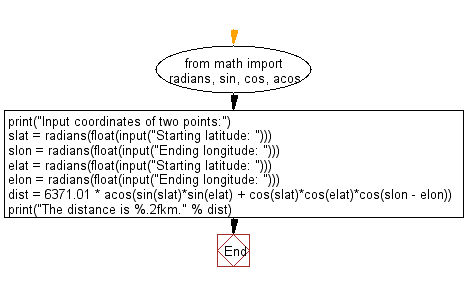﻿ Python Math: Distance between two points using latitude and longitude - w3resource# Python Math: Distance between two points using latitude and longitude

## Python Math: Exercise-27 with Solution

Write a Python program to calculate distance between two points using latitude and longitude.

Sample Solution:-

Python Code:

``````from math import radians, sin, cos, acos

print("Input coordinates of two points:")

dist = 6371.01 * acos(sin(slat)*sin(elat) + cos(slat)*cos(elat)*cos(slon - elon))
print("The distance is %.2fkm." % dist)
```
```

Sample Output:

```Input coordinates of two points:
Starting latitude: 23.5
Ending longitude: 67.5
Starting latitude: 25.5
Ending longitude: 69.5
The distance is 300.67km.
```

Flowchart:## Visualize Python code execution:

The following tool visualize what the computer is doing step-by-step as it executes the said program:

Python Code Editor:

Have another way to solve this solution? Contribute your code (and comments) through Disqus.

What is the difficulty level of this exercise?

Test your Programming skills with w3resource's quiz.

﻿

```>>> students = [{'name': 'John', 'score': 98}, {'name': 'Mike', 'score': 94}, {'name': 'Jennifer', 'score': 99}]